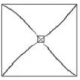Douglas Larsson Engholm and the Master Builders Grid and 137

The following blog post highlights the fine inspired work of Douglas Larsson Engholm.
This is his blog here called Ride the Tide

Douglas seems to updating his blog daily with his brain fARTS that seem to be aligning with a cosmic heART pattern … this is all good … Douglas and ME+me are driven by a similar passion.

So if phi and pi and 137 and Pitagoras’ right angle theorem all hold some fascination for you … consider Doug’s blog and the one you currently are on are both in support of an idea whose time has come i.e. defining broken symmetry using the 2D chiral swastika .. simply because what Douglas and ME+me are proposing address ‘what time is’ … maybe even helping us answer the big question;

What is consciousness … what is spooky action at a distance … what is entanglement … are they one and the same?

Some time ago Douglas sent ME+me these images … starting with Step 1 and Step 2.Important to note how the entire process begins with a unit of 1 and ends up with an area of 137 by the time we arrive at Step 15.However in order to make the above image comprehensible to me … I needed to add the following dimensions to his sketch.
Aha … a clue to why and how the ancients decided the integer 137 held some significance.I then asked Doug if he could make a video of the transformation that begins with a simple algorithm.

And he did.

A shifting mathematical structure to derive numbers using Pythagoras theorem.

All of these drawings are completely mathematical, even though some seems artistic every point in these drawings are mathematical points.
Here is the swastika with a bounding box and with added hypotenuses to use the Pythagorean theorem. The theorem describe the effect when these angles move.
Also note the square and the circle in the middle and see their corresponding numbers you will see that they have equal circumference and area down to 6 decimals at one point as they are growing through each other. That was all that was possible at this moment in this program.

– Douglas Larsson Engholm

ALL of Douglas’ videos can be found here;

Some can be found in the following links …

Shifting proof of Pythagoras Theorem

If you draw a diamond square where each corner of the square is attached to the end of the long leg of each angle. What you will get when you start separating the angles is a proof of Pythagoras theorem for every possible triangle with a hypotenuse from root square 2 to root square 5 ,regardless of what unit you use. That means it is a numberless proof for the pythagorean theorem.
You also get a very beautiful square spiral.

Squaring the circle

Is it possible to do the impossible? Let’s draw a square where the corners is attached to the long leg of each angle, and then draw a circle which is attached to the 90 degree corner of each angle. From the beginning the circle surrounds the square, but as the transformation occurs and the angles start separating the relationship between the square and the circle changes. They are passing through each other, as the circle diminishes in size and the square grows, though still sharing the same center point. It is like two people meet on the same step as one is going up the stairs and the other one is going down, they are bound to meet somewhere, no? Meaning that at one point their circumference and area should be exactly equal. They meet in infinity.

Zero to 3 dimensions

From one single dot (0 D) to a cubic structure (3 D). By starting with the function of pi through to Phi and then the swastika and continuing to the cubic unit with 3 planes intersecting. Showing how to build the field from one single point.
This is just one unit but all are constructed the same.

2B continued …

Take note of the square root spiral.•Douglas Engholm  On September 18, 2019 at 1:44 pm